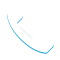### Advanced Statistical Analysis Using IBM SPSS Statistics (V25) – 0G09AG CR

Details

Course Code: 0G09AG

Brand: DS&BA – SPSS

Category: Analytics

Duration: 16.0H

Modality: CR

Audience

Anyone who works with IBM SPSS Statistics and wants to learn advanced statistical procedures to be able to better answer research questions.

Prerequisites

• Experience with IBM SPSS Statistics (navigation through windows; using dialog boxes)
• Knowledge of statistics, either by on the job experience, intermediate-level statistics oriented courses, or completion of the Statistical Analysis Using IBM SPSS Statistics (V25) course.

Short Summary

In this course you will learn how to use advanced statistical procedures, such as Factor, Cluster, Decision Trees, Cox Regression, and Linear Mixed models, using IBM SPSS Statistics (V25).

Overview

This course provides an application-oriented introduction to advanced statistical methods available in IBM SPSS Statistics. Students will review a variety of advanced statistical techniques and discuss situations in which each technique would be used, the assumptions made by each method, how to set up the analysis, and how to interpret the results. This includes a broad range of techniques for predicting variables, as well as methods to cluster variables and cases.

Topic

• Taxonomy of models
• Overview of supervised models
• Overview of models to create natural groupings

Group variables: Factor Analysis and Principal Components Analysis
• Factor Analysis basics
• Principal Components basics
• Assumptions of Factor Analysis
• Key issues in Factor Analysis
• Improve the interpretability
• Use Factor and component scores

Group similar cases: Cluster Analysis
• Cluster Analysis basics
• Key issues in Cluster Analysis
• K-Means Cluster Analysis
• Assumptions of K-Means Cluster Analysis
• TwoStep Cluster Analysis
• Assumptions of TwoStep Cluster Analysis

Predict categorical targets with Nearest Neighbor Analysis
• Nearest Neighbor Analysis basics
• Key issues in Nearest Neighbor Analysis
• Assess model fit

Predict categorical targets with Discriminant Analysis
• Discriminant Analysis basics
• The Discriminant Analysis model
• Core concepts of Discriminant Analysis
• Classification of cases
• Assumptions of Discriminant Analysis
• Validate the solution

Predict categorical targets with Logistic Regression
• Binary Logistic Regression basics
• The Binary Logistic Regression model
• Multinomial Logistic Regression basics
• Assumptions of Logistic Regression procedures
• Testing hypotheses

Predict categorical targets with Decision Trees
• Decision Trees basics
• Validate the solution
• Explore CHAID
• Explore CRT
• Comparing Decision Trees methods

Introduction to Survival Analysis
• Survival Analysis basics
• Kaplan-Meier Analysis
• Assumptions of Kaplan-Meier Analysis
• Cox Regression
• Assumptions of Cox Regression

Introduction to Generalized Linear Models
• Generalized Linear Models basics
• Available distributions

Introduction to Linear Mixed Models
• Linear Mixed Models basics
• Hierachical Linear Models
• Modeling strategy
• Assumptions of Linear Mixed Models

Objectives

• Introduction to advanced statistical analysis
• Group variables: Factor Analysis and Principal Components Analysis
• Group similar cases: Cluster Analysis
• Predict categorical targets with Nearest Neighbor Analysis
• Predict categorical targets with Discriminant Analysis
• Predict categorical targets with Logistic Regression
• Predict categorical targets with Decision Trees
• Introduction to Survival Analysis
• Introduction to Generalized Linear Models
• Introduction to Linear Mixed Models
Tags :Call Now +27 72 266 2599